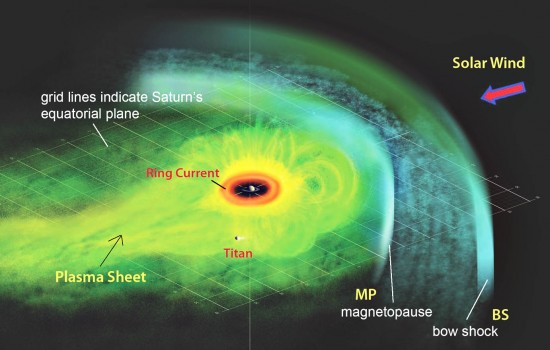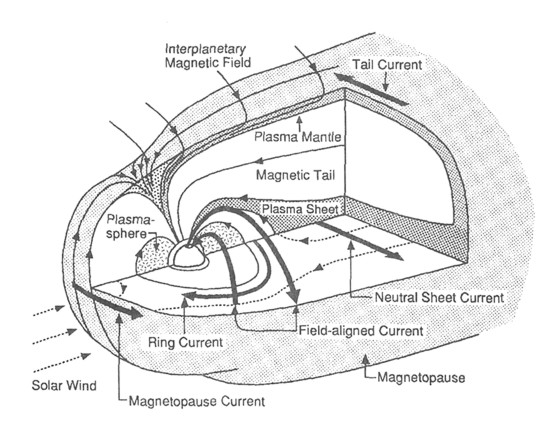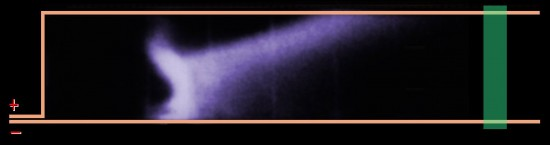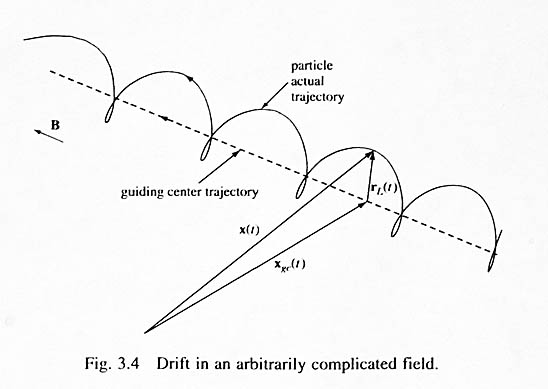# Essential Guide to the EU – Chapter 8Current Sheets, Perpendicular Currents and Electric Circuits

## 8.1  Plasma Current Sheets

Reference has already been made to the filamentation of current sheets. This section will explore the nature of current sheets and their relationship to the magnetic field.Artist's interpretation of Saturn's plasma sheet, based on data from Cassini's Magnetospheric Imaging Instrument.The plasma sheet, separating the upper and lower magnetosphere halves, thins gradually toward the nightside of the planet. The magnetopause indicates the flow of the deflected solar wind. Credit: NASA/Jet Propulsion Laboratory / Johns Hopkins University Applied Physics Laboratory

A current sheet is exactly what it says – a thin surface within which a current flows. It obviously differs from a diffuse cloud of moving charge and from a cylindrical filament of current. A current sheet forms a surface between two regions of plasma, somewhat like a Double Layer, and, also like a DL, often separates regions of different characteristics.

The current in the sheet flows in one direction, contained entirely within the sheet. One can think of it as though the current was flowing in the warp threads of a woven linen sheet: all the current flows in the same direction in each of the warp threads, and no current flows in the weft threads. A current, of course, consists of ions and electrons flowing in opposite directions, so the current sheet will contain both types of particle.

Obviously the direction of the current can change as the sheet itself does not need to be planar. For example, there is clear evidence of a part-spherical current sheet at the ‘bow shock’ where the Earth’s magnetosphere interacts with the incoming solar wind.Cutaway sketch of Earth's plasma current sheets in and around its magnetosphere. Image credit: Wikipedia Images, numerous websites without attribution source

If we analyze the magnetic field near a current sheet we find that the magnetic force due to the current sheet is in opposite directions on either side of the sheet. For example, if a current is flowing up this page, then above the page the magnetic field will be from left to right, and below the page it will be from right to left, as one would expect from the rotation right-hand rule for any individual ‘thread’ of current. (Note that the rotation right hand rule is not the same as the vector cross-product right hand rule !)

Thus a principal effect of a current sheet is to create separate areas of opposing magnetic fields. At the sheet location itself, the magnetic field is zero. This is exactly the situation that has been found in the tail area of the Earth’s magnetosphere, where a current sheet in the equatorial plane separates zones of opposing magnetic field. In this case, the tail sheet current flows azimuthally or ‘west to east’ and the magnetic fields are radial, being aligned towards the Earth in the Northern hemisphere and away from the Earth in the Southern hemisphere.

The Gravity Model describes these current sheets as caused by the opposing magnetic fields on either side. Remembering that magnetic fields are force fields that are caused by the motion of charged particles, that is, currents, the Gravity Model appears to be inverting cause and effect. What the magnetic fields created by the current actually do is to compress the current into the form of a sheet. They do not create the current.

Current sheets therefore are another means by which plasma can cellularize in response to differing environments.

Current sheets can also act to accelerate mass in a pulsed plasma thruster application.  See text and video from Princeton University’s Electric Propulsion and Plasma Physics Lab here.## 8.2  Perpendicular Currents

We have already considered the cases where currents flow parallel to (are “field-aligned with”) the magnetic field (filaments and Birkeland Currents) and the case where currents flow in regions of zero field (current sheets). The remaining possibility is for currents to have a vector component that is perpendicular to the magnetic field under the influence of nonmagnetic forces acting in combination with the magnetic field (see 8.3 below).

[Recall that F is the resulting force vector on a charged particle; q is the value of the amount of charge on the particle; E is the electric field’s vector value at a specified time and coordinate; U is the velocity vector of the charged particle at that time and coordinate, and B is the magnetic field vector at that time and coordinate.  Finally, note that the bold vectors refer to a scalar magnitude value plus a direction, e.g., 3000 km/s heading east.]

The Lorentz Force on a charged particle, F = q(E + U × B) in vector algebra, depends on the relationship of the velocity of the particle U to the magnetic field B. The magnitude of the vector cross product U × B can be written UB sin θ, where θ is the smaller angle between U and B.  The direction of the force produced by U × B is given by the movement of a right-handed screw turned from U to B, that is, it is at right angles to both U and B.

This causes a charged particle moving at right angles to the magnetic field to follow a circular path in a plane perpendicular to the field. We can call this the centripetal force. If E is nonzero, the particle will also accelerate in the direction of E.

Obviously, if U is zero or parallel to B then there is no centripetal force on the particle from the magnetic field. In other words, if the particle is stationary or moving parallel to the field, then it will experience no magnetic force.

Rather than considering variable angles between U and B, it is easier to consider the parallel and perpendicular components of U separately. As only the perpendicular component causes a force, we shall concentrate on that component alone. We shall also assume E = 0 unless stated otherwise.

The particle velocity that would result from a combination of a force-induced motion and a magnetic field can be considered as a circular motion around a guiding center (image below), which center itself drifts perpendicular to the magnetic field with velocity vp given by the Guiding Center Equation:

vp = (F × B) / qB2

Note that F is any nonmagnetic force (for example, gravity, or an electric field) which causes motion of a charged particle. This motion then interacts with the magnetic field according to Lorentz’s Law. When B is in the z direction and F is in the y direction in Cartesian coordinates, then the resulting velocity vp is in the x direction.Helical trajectory of a charged particle, with its circular motion superimposed on its drift velocity vector. Image credit: "Fundamentals of Plasma Physics", Cambridge Press, 2006; Dr. Paul Bellan, California Institute of Technology

What this equation tells us is that, when a particle is subject to an external force perpendicular to the magnetic field, it will acquire a constant velocity vp perpendicular to both the field and the force. How this comes about is as follows:

If a particle is initially at rest, an external force (an electric field, say) will start to accelerate it in the direction of the force according to Newton’s laws. However, as soon as the particle acquires some small amount of velocity or velocity component perpendicular to the magnetic field, then a centripetal force emerges as a result of the magnetic field and starts to turn the path of the particle away from the trajectory induced by the external force.

The external force is still trying to accelerate the particle in the direction of the force, but there is now a component of the centripetal force which opposes the external force. The acceleration in the direction of the external force will be reduced accordingly.

Under the influence of the twin external and centripetal forces, the particle will follow a curved path turning through 90 degrees. At the point when the path is perpendicular to the external force, the particle has acquired the velocity vp given by the Guiding Center Equation, and the centripetal force due to the interaction of vp and B exactly balances the external force.

Therefore, there will be no more acceleration in the direction of the external force, nor will there be any acceleration in the direction of vp because there is no force in that direction. The particle has acquired a constant velocity perpendicular to both B and the external force.

As long as the particle continues to move with velocity vp in the perpendicular direction, then the situation is stable, and the external force remains balanced by the centripetal force.

## 8.3  Effect of Various External Forces

The above discussion applies to any constant external force acting on a charged particle in a magnetic field. Various forces can cause velocities in the direction perpendicular to the magnetic field. These include gravity, an electric field, and inertial forces. Each will have a different effect dependent on whether the external force is a function of the mass of, or the charge on, the particle, as follows:

Case A.  Electrical Field Force, FE × B for an electric field perpendicular to B.

Because FE = qE, the Guiding Center Equation becomes:

vp = (E x B) / B2

• In Case A, the perpendicular velocity is independent of the charge on the particle. This results in the special case of ions and electrons both drifting in the same direction, as we saw when considering the concentration of matter by filamentary currents.

Case B.  Gravity, Fg×B

Because Fg = mg , the resulting perpendicular drift velocity is dependent on both the mass of the particles and their charge, and for Case B:

vp = (g × B) × m/qB²

• Ions and electrons will therefore move in opposite directions, resulting in a current, charge separation, and zones of different potential (i.e., electric fields). All these effects will occur simply as a result of the interaction of gravity and a magnetic field. Obviously these effects will then start to cause secondary effects of their own, and complex plasma behavior can result. (Ref: Fundamentals of Cosmic Electrodynamics, Boris V. Somov, Kluwer Academic Publishers, 1994, Chapter 2, Motion of a Charged Particle in Given Fields)
• In addition, the dependence of the velocity on the mass of the particles can also result in chemical separation of different ions, or Marklund Convection.
• One case in particular is of interest here. Consider the Earth and its magnetic field, which can be visualized as field lines spreading out into near space arranged somewhat like the segments of an orange. In the equatorial plane, the field will be aligned north-south. The gravitational force will be radially inwards and so at right angles to the field.
• Any ions and electrons in the vicinity, for example, in the ionosphere, will therefore acquire velocities perpendicular to both B and g under the combined influence of gravity and the magnetic field. Because the velocities of ions and electrons are in opposite directions, this is equivalent to a current flowing in a ring around the equatorial plane. The Van Allen belts are examples of ring currents.

• This is an inevitable result of the presence of charged particles in a magnetic field orientated at right angles to the gravitational field. A current will always be generated in this situation.  Several of the moons of Jupiter and Saturn exhibit these currents, evidenced by the electromagnetic radiation where the induced currents come in contact with the planets’ atmospheres in the vicinity of their polar auroral ovals.

Case C. Inertia, Fi = -m (du/dt)      (Newton’s Second Law of Motion)

In this case the charged particles already have an initial momentum mu (inertial mass times the velocity vector) when they encounter a magnetic field. The Guiding Center Equation indicates that the initial momentum will be altered by the magnetic field:

vp = -mq/B² du/dt × B

As vp is charge-dependent, the final velocity of ions and electrons is in opposite directions and therefore represents a current. Ions of different masses will acquire different final velocities and so will be chemically sorted. There is also another important effect of inertial effects:

• If a volume of plasma is accelerated to a particular velocity due to, for example, an I × B force in the region (which accelerates oppositely moving ions and electrons in the same perpendicular direction), then the plasma has acquired kinetic energy at the expense of the circuit driving the current.
• If this volume of moving plasma then enters another region where it can establish a circuit in the local plasma, its velocity vp will cause a current perpendicular to both B and vp . The interaction of this current with B will cause a force on the moving plasma which slows it down. In other words, the plasma’s kinetic energy is given up again in generating a current in a new location.
• Therefore the interaction of the inertial motion of charged particles and a magnetic field is a means by which kinetic energy can be exchanged with electromagnetic energy, and therefore it is a means by which energy can be transported between different locations.

## 8.4  Electrical Circuits in Plasma

Unless charge is flowing from an electrostatic source or to a sink, then it will form part of a closed circuit. In space, the circuit may not always be obvious because the conductors are often invisible and may close the circuit at vast distances from the areas of interest, but they must close somewhere.

Consideration of the circuits in space can explain behavior such as transport of energy from one area to another which drives detectable electrical activity in a region under investigation.

In this context, it is worth pointing out that if a plasma containing any regions of slight charge imbalance is moving relative to another region of plasma in a magnetic field, then the first region will induce an electric field and currents in the second region due to the interaction of the electromagnetic fields and forces.

The Gravity Model holds that Debye screening, which is due to similar effects as cause a Debye sheath around a charged body, limits the extent of charge imbalances to the Debye length. However, the v × B force from the Lorentz equation is independent of the Debye length and can induce an electric field in another region of plasma well beyond the Debye limit.

## 8.5  Double Layers as Circuit Elements

Any Double Layer accelerates ions and electrons due to the potential drop across the DL. If the DL is a current-carrying DL, then it effectively forms part of an electrical circuit in which the current is flowing. The energy to accelerate the particles is supplied by the circuit and converted within the DL into kinetic energy.

The DL therefore acts as an inertial resistance and may experience a reaction which causes its position to drift. This is analogous to the recoil of a gun as its power source accelerates the mass of the bullet. Particles accelerated by the DL cause a pressure on the surrounding plasma, with which they interact and cause radiation. Dissipation of excess energy in this fashion can allow the plasma to reach a stable state via the formation of a DL which provides the necessary mechanism.

## 8.6  Energy and Inductance

The circuit energy supplied to the DL may originate in the energy stored in the magnetic field or in the kinetic energy of the bulk plasma. In circuit terms, an element which stores energy is an inductor. The plasma may therefore be thought of as analogous to an inductor in a simple circuit. Similarly, the DL behaves in some respects as a capacitor, although one with variable characteristics, including a resistance which can reduce with increasing current.

All electrical circuits which have inductance are potentially unstable, depending on the values of voltage, inductance, resistance, and capacitance around the circuit. If the total resistance of the circuit is negative, which is often the case in plasma because of the falling characteristic of the I-V (current versus voltage) curve, then stability of the inductive circuit is impossible. A simple circuit involving voltage, inductance, and negative resistance will either oscillate or dissipate all its energy and become extinct.

If the potential drop across the DL is larger than the plasma potential, then the DL is classified as a strong DL. A strong DL will reflect particles that approach the DL with energies less than the plasma potential. Only those particles with energies above the plasma potential will enter the DL and be accelerated by its voltage differential, i.e., electric field.

The behavior of plasma in a CCDL is thus dependent on the characteristics of the external circuit which is driving the formation of the CCDL.

## 8.7  Resonant Circuits

A circuit containing inductance and capacitance has a natural or resonant frequency at which it will oscillate electrically. Similarly, a plasma circuit containing inductance in the form of stored magnetic energy and a CCDL exhibiting negative resistance will tend to have a resonant frequency at which energy is exchanged between the electric field in the DL and the magnetic field in the plasma. As the electric field in the DL increases, it will accelerate particles to higher energies in the normal manner.

It is apparent that this model is an efficient means of generating high-frequency bursts of radiation. By contrast, the Gravity Model postulates very high density neutron stars rotating up to thousands of times a second in order to explain this commonly-observed phenomenon.

Not all situations result in a resonant frequency. Variations often result in oscillations over a wide frequency band. The DL is then ‘noisy’ in electrical circuit terms. The effect of the noise is to create a range of electron energies in the beam accelerated by the DL. Some electrons then have enough energy to break out of the magnetic field confining the current, and this can lead to expansion of the plasma.

End of Chapter 8